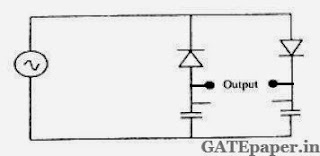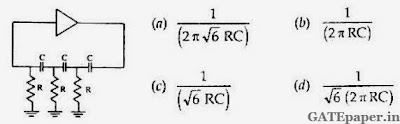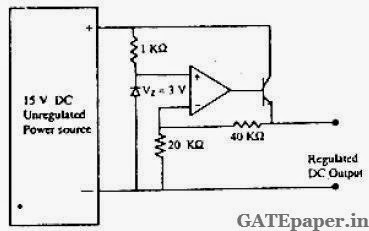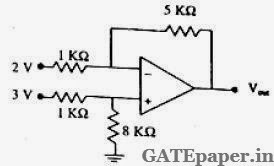### GATE 2003 ECE Video Solutions on Analog Circuits (Analog Electronics)

1. Choose the correct match of input resistance of various amplifier configurations shown below:2. The circuit shown in the figure is best described as aa. Bridge rectifier
b. Ring modulator
c. Frequency discriminator
d. Voltage doubler

3. If the input to the ideal comparator shown in the figure is a sinusoidal signal of 8 volts peak to peak, without any DC component, then the output of the comparator has a duty cycle ofa. 1/2
b. 1/3
c. 1/6
d. 1/2

4. If the differential voltage gain and the common mode voltage gain of a differential amplifier are 48 dB and 2 dB respectively, then common mode rejection ratio is
a. 23 dB
b. 25 dB
c. 46 dB
d. 50 dB

5. Generally, the gain of a transistor amplifier falls at high frequencies due to the
a. Internal capacitance of the device
b. Coupling capacitor at the input
c. Skin effect
d. Coupling capacitor at the output

6. An amplifier without feedback has a voltage gain of 50, input resistance of 1 KΩ and output resistance of 2.5 KΩ. The input resistance of the current-shunt negative feedback amplifier using the above amplifier with a feedback factor of 0.2 is
a. 1/11 KΩ
b. 1/5 KΩ
c. 5 KΩ
d. 11 KΩ

7. In the amplifier circuit shown in the figure, the values of R1 and R2 are such that the transistor is operating at VCE = 3 volts and IC = 1.5 mA, when its β is 150.for a transistor with β of 200, the operating point (VCE, IC) isa. (2 volts, 2 mA)
b. (3 volts, 2 mA)
c. (4 volts, 2 mA)
d. (4 volts, 1 mA)

8. The oscillator circuit shown in the figure has an ideal inverting amplifier. Its frequency of oscillation in Hertz is9. The output voltage of the regulated power supply shown in the figure isa. 3 volts
b. 6 volts
c. 9 volts
d. 12 volts

10. If the OP-AMP in the figure is ideal, the output voltage VOUT will be equal toa. 1 volt
b. 6 volts
c. 14 volts
d. 17 volts

11. Three identical amplifiers with each one having a voltage gain of 50, input resistance of 1 KΩ and output resistance of 250 Ω are cascaded. The open circuit voltage gain of the combined amplifier is
a. 49 dB
b. 51 dB
c. 98 dB
d. 102 dB

12. An ideal saw-tooth voltages waveform of frequency of 500 Hz and amplitude 3 volts is generated by charging a capacitor of 2 µF in every cycle. The charging requires
a. Constant voltage source of 3 volts for 1 ms
b. Constant voltage source of 3 volts for 2 ms
c. Constant current source of 1 mA for 1 ms
d. Constant current source of 3 mA for 2 ms